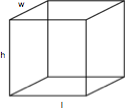## ASVAB Math Knowledge Solids Practice Test 402952

Question 1 of 5
###### Cubes

A cube is a rectangular solid box with a height (h), length (l), and width (w). The volume is h x l x w and the surface area is 2lw x 2wh + 2lh.The dimensions of this cube are height (h) = 1, length (l) = 1, and width (w) = 5. What is the surface area?

 88 22 292 120